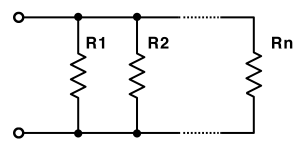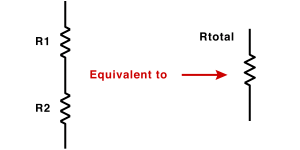# Parallel and Series Resistor Calculator

This tool calculates the overall resistance value for multiple resistances connected either in series or in parallel.

### Parallel Resistor

R1
R2
R3
R4
R5
R6
R7
R8
R9
R10
Total Parallel Resistance
=

### Series Resistor

R1
R2
R3
R4
R5
R6
R7
R8
R9
R10
Total Series Resistance
=

## Calculate the total parallel resistance

Note: You can increase the number of resistors for multiple resistance connected in series or parallel.

## Calculate the total series resistance

Note: You can increase the number of resistors for multiple resistance connected in series or parallel.×